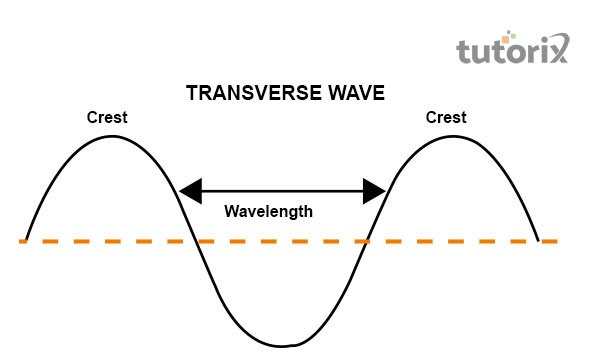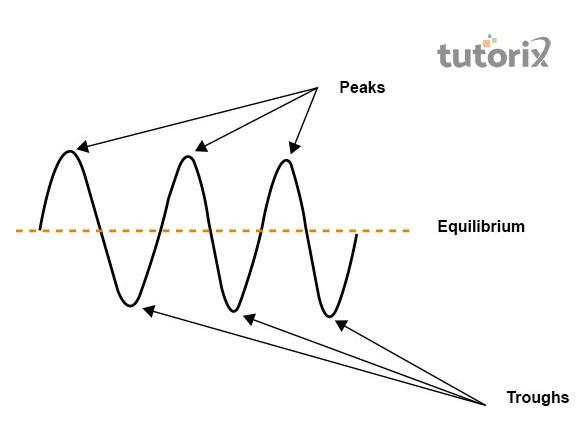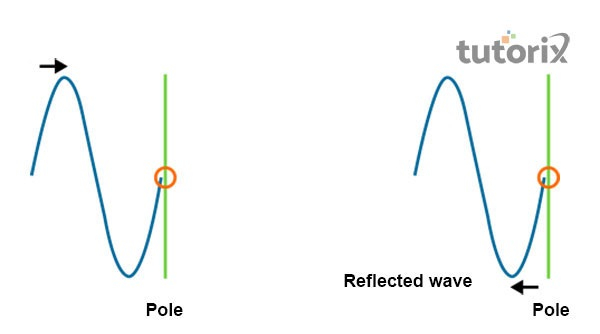# Transverse Waves

## Introduction

Transverse wave mainly refers to those waves in which the displacement or disturbance of direction acts as a medium perpendicular to that of the propagation of wave. It is seen that waves are present everywhere and they can be described as disturbances and they flow from one place to another place through the support of a medium. The transverse waves consist of some characteristics and different kinds of waves in this field can be seen. Among the different characteristics, it is said that waves can only pass through solids.

## Definition of Transverse Wave

Transverse waves are the kind of waves in which the vibration moves in a direction perpendicular to the direction of the propagation of the wave. The waves in the water are a great example of that kind of wave. It is seen that when the waves move linearly, the particles are not moving. It is seen that in this kind of wave progress is observed through a series of crests and troughs.

The distance between the crests is called the wavelength. The main characteristic of this wave is that it happens only in that case of solid particles. It does not have the capacity to happen through the medium of gas. The perpendicular motion is very important in this kind of wavelength.Figure 1: Transverse Wave

## Examples of Transverse Wave

There are various kinds of examples of the transverse wave and they are very useful for understanding the concept of physics. Ocean waves are the most common example of this and another example can be a stadium or a human wave. An earthquake’s secondary waves and the electromagnetic waves are also examples of transverse waves.

## Speed of Transverse Wave

The speed of the transverse wave can be measured through the troughs and peaks of this matter. In this part, the former means the bottom point of the wave whereas peaks mean the top point (Weberg, Morton & McLaughlin, 2020). It is seen that the wave moves from the left side to the right side. The measuring of speed depends upon some factors like wavelength, frequency, amplitude, and period. Here wavelength refers to the distance between one peak and the next peak.

Frequency refers to the number of wavelengths which travels through a certain point in a second. Amplitude is an important factor here and it is the maximum displacement of a particle from its equilibrium position. Period means the time it takes for covering two successive peaks for passing through a fixed point (Jong et al. 2019). It is seen that speed mainly depends upon two factors. The first one is wave and the latter is the object through which it passes.Figure 2: Speed of Transverse Wave

## Calculation of this method

The velocity of a wave can be determined by multiplying the distance travelled by the time. This is calculated through the division of wavelength with time. It is: $\mathrm{v\:=\:\lambda/T}$.

The proportional relationship is described through the formula of: $\mathrm{v\:=\:\sqrt{(T/\mu)}}$.

## Reflection of this wave

The reflection of a transverse wave depends upon the matter of fixing the point of the wave. Before going into the matter it is important to note whether the waves can be fixed to both ends or not.Figure 3: Reflection of Transverse wave

The image here shows the reflection of a transverse wave and it is reflected from a fixed end. It is seen that during the time of meeting a fixed end, transverse wave is reflected but it is also inverted. This matter shows the peak point and the bottom point of the wave. On the other hand, another image shows that transverse wave on a string and it meets a free end. In this part, the wave is reflected but it is not inverted to the fixed end like the former one. This is a matter of reflection on this kind of wave.

## Conclusion

The concept of transverse wave says that it is the kind of wave and it passes through the solid medium. It does not have the ability to pass through liquid or gases. The phenomenon of polarisation can be observed in the case of transverse wave. The most common example of this kind of wave is the ocean waves. It mainly defines the flow of energy in the form of vibration. The moving of the vibration is seen in the direction of perpendicular and it goes to the direction of propagation of the wave. The wave shows that the particles do not move for giving the measure of the waves.

## FAQs

Q1. Does the speed of the wave affect through Tension?

Ans. Yes, the tension affects the wave of speed and with the incensement of tension in a string, the wave’s speed increases. The frequency is also increased and it affects the speed of the wave.

Q2. How can the transverse wave form?

Ans. It is seen that when the perpendicular motion is directed towards the wave’s propagation then it is triggered in the molecule and here transverse wave is created. The molecules help in the creation of high and low peaks of the wave.

Q3. What are the important terminologies for the speed of the transverse wave?

Ans. There are some important terminologies for the measurement of the speed of the transverse wave. They are- frequency, period, wavelength, and amplitude. Frequency refers to the number of wavelengths and wavelength is the distance of peaks in the wave in this part of the tutorial.

Q4. What are the examples of transverse waves?

Ans. Radio waves, light waves, and different electromagnetic waves are common examples of the transverse wave. This kind of wave is formed on the surface of the water and it is seen that the water molecules travel up and down from their mean position in water.

Updated on: 13-Oct-2022

428 Views